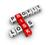# Profit & LossProfit and Loss Quantitative Aptitude Questions and Answers with video solution and explanation for competitive examinations. Each question is followed by a video solution for better understanding and practice.

# The total cost of 8 buckets and 5 mugs is 92 Rs. and the total cost of 5 buckets and 8 mugs is 77 Rs. Find the cost of 2 mugs and 3 buckets.

The total cost of 8 buckets and 5 mugs is 92 Rs. and the total cost of 5 buckets and 8 mugs is 77 Rs. Find the cost of 2 mugs and 3 buckets. [A]30 Rs. [B]35 Rs. [C]38 Rs. [D]70 Rs. Show Answer 35 Rs. C.P. of 1 bucket = x C.P. of 1 ..

# If a shirt costs 64 Rs. After 20% is allowed, what was its original price?

If a shirt costs 64 Rs. After 20% is allowed, what was its original price? [A]76.80 Rs. [B]80 Rs. [C]86.80 Rs. [D]88 Rs. Show Answer 80 Rs. If the original cost of shirt be x, then $latex x\times \frac{80}{100} = 64&s=1$ $latex => x = \frac{64\times 100}{80} = 80 Rs.&s=1$ Hence option [B] is the ..

# The cost price of a radio is 600 Rs. The 5% of the cost price is charged towards transportation. After adding that, if the net profit to be made is 15%, then the selling price of the radio must be :

The cost price of a radio is 600 Rs. The 5% of the cost price is charged towards transportation. After adding that, if the net profit to be made is 15%, then the selling price of the radio must be : [A]664.50 Rs. [B]684.50 Rs. [C]704.50 Rs. [D]724.50 Rs. Show Answer 724.50 Rs. Actual C.P. ..

# A merchant purchases a wrist watch for 450 Rs. and fixes its list price in such a way that after allowing a discount of 10%, he earns a profit of 20%. Then the list price of the watch is :

A merchant purchases a wrist watch for 450 Rs. and fixes its list price in such a way that after allowing a discount of 10%, he earns a profit of 20%. Then the list price of the watch is : [A]550 Rs. [B]600 Rs. [C]650 Rs. [D]700 Rs. Show Answer 600 Rs. If the marked ..

# While selling to the retailer, a company allows 30% discount on the marked price on their products. If the retailer sells those products at marked price, his profit % will be:

While selling to the retailer, a company allows 30% discount on the marked price on their products. If the retailer sells those products at marked price, his profit % will be: [A]$latex 30\%$ [B]$latex 40\%$ [C]$latex 42\frac{6}{7}\%&s=1$ [D]$latex 42\frac{1}{7}\%&s=1$ Show Answer $latex \mathbf{42\frac{6}{7}\%}&s=1$ If the marked price of the product be 100 Rs., then C.P. ..